DFT (Frequency Domain Sampling) - Digital Signal Processing, Engineering

# DFT (Frequency Domain Sampling) - Digital Signal Processing, Engineering Notes - Computer Science Engineering (CSE)

## Document Description: DFT (Frequency Domain Sampling) - Digital Signal Processing, Engineering for Computer Science Engineering (CSE) 2022 is part of Computer Science Engineering (CSE) preparation. The notes and questions for DFT (Frequency Domain Sampling) - Digital Signal Processing, Engineering have been prepared according to the Computer Science Engineering (CSE) exam syllabus. Information about DFT (Frequency Domain Sampling) - Digital Signal Processing, Engineering covers topics like and DFT (Frequency Domain Sampling) - Digital Signal Processing, Engineering Example, for Computer Science Engineering (CSE) 2022 Exam. Find important definitions, questions, notes, meanings, examples, exercises and tests below for DFT (Frequency Domain Sampling) - Digital Signal Processing, Engineering.

Introduction of DFT (Frequency Domain Sampling) - Digital Signal Processing, Engineering in English is available as part of our Computer Science Engineering (CSE) preparation & DFT (Frequency Domain Sampling) - Digital Signal Processing, Engineering in Hindi for Computer Science Engineering (CSE) courses. Download more important topics, notes, lectures and mock test series for Computer Science Engineering (CSE) Exam by signing up for free. Computer Science Engineering (CSE): DFT (Frequency Domain Sampling) - Digital Signal Processing, Engineering Notes - Computer Science Engineering (CSE)
 1 Crore+ students have signed up on EduRev. Have you?

DFT (Frequency Domain Sampling)

The Fourier series describes periodic signals by discrete spectra, where as the DTFT describes discrete signals by periodic spectra. These results are a consequence of the fact that sampling on domain induces periodic extension in the other. As a result, signals that are both discrete and periodic in one domain are also periodic and discrete in the other. This is the basis for the formulation of the DFT.

Consider aperiodic discrete time signal x (n) with FT X(w) =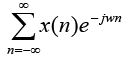Since X (w) is periodic with period 2π , sample X(w) periodically with N equidistance samples with spacing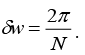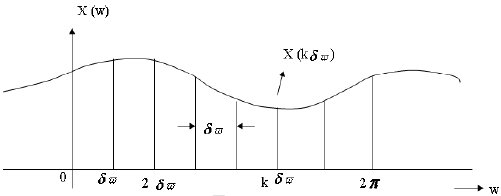K = 0, 1, 2…..N-1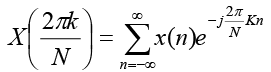The summation can be subdivided into an infinite no. of summations, where each sum
contains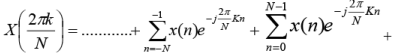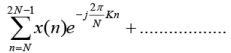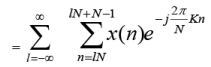Put n = n-lN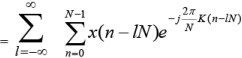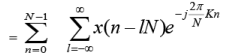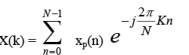We know that xp(n) =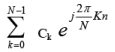n= 0 to N-1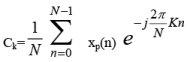k=0 to N-1

Therefore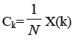k=0 to N-1

DFT ------------ xp (n) =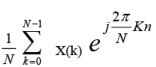n = 0 to N-1

This provides the reconstruction of periodic signal xp(n) from the samples of spectrum
X(w).

The spectrum of aperiodic discrete time signal with finite duration L<N, can be exactly

recovered from its samples at frequency Wk=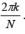Prove: x(n) = xp (n) 0 ≤ n ≤ N-1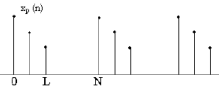Using IDFT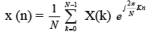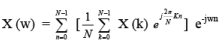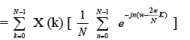If we define    p(w) =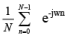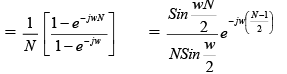Therefore: X (w) =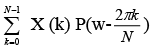At w =2πk/N P (0) =1

And P (w 2πk/N)= 0 for all other values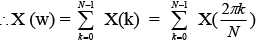Ex: x(n) = an u(n) 0<a<1

The spectrum of this signal is sampled at frequency Wk=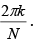k=0, 1…..N-1, determine reconstructed spectra for a = 0.8 and N = 5 & 50.

X (w) =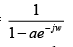X (wk) =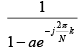k=0, 1, 2… N-1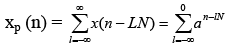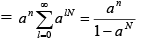0≤n≤N-1

Aliasing effects are negligible for N=50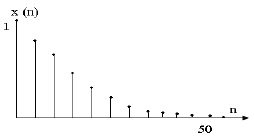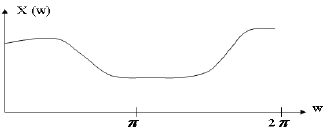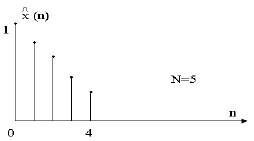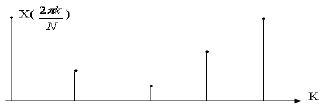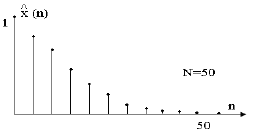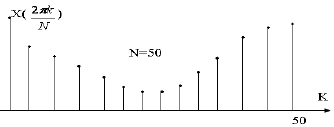If we define aliased finite duration sequence x(n)

xˆ(n)= xp(n)        0≤n≤N-1

= 0                  otherwise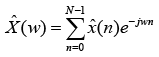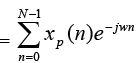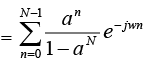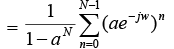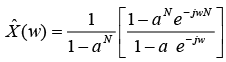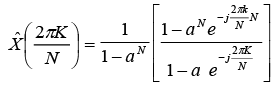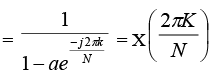∴Although XÆ (w) ≠ X (w), the samples at Wk=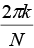are identical.

Ex: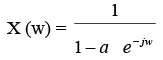&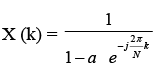Apply IDFT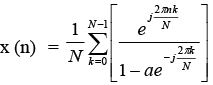using Taylor series expansion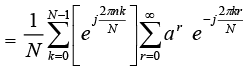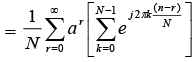= 0  except r = n+mN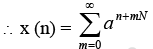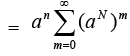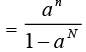The result is not equal to x (n), although it approaches x (m) as N becomes ∞ .

Ex: x (n) = {0, 1, 2, 3} find X (k) =?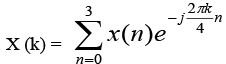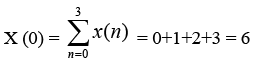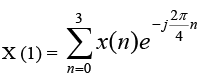= -2+2j

X (2) = -2
X (3) = -2-2j

DFT as a linear transformation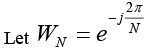k = 0 to N-1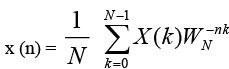n = 0, 1…N-1

Let xN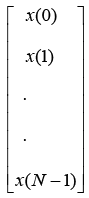XN =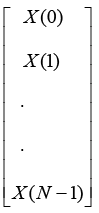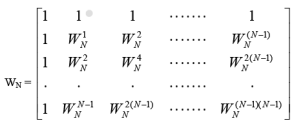The N point DFT may be expressed in matrix form as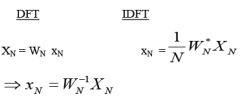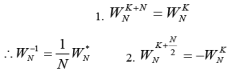Ex: x (n) = {0, 1, 2, 3}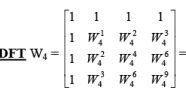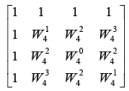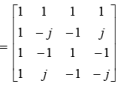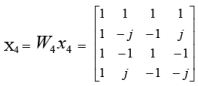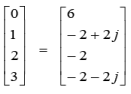IDFT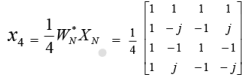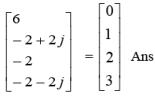Q.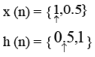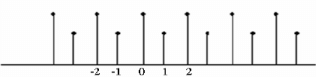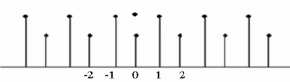Find y (n) = x (n) h (n) using frequency domain. Since y (n) is periodic with period 2.
Find 2-point DFT of each sequence.

X (0) = 1.5 H (0) = 1.5

X (1) = 0.5    H (1) = -0.5

Y (K) = X (K) H (K)

Y (0) = 2.25 Y (1) = -0.25

Using IDFT  y (0) = 1; y (1) = 1.25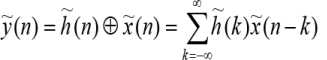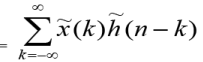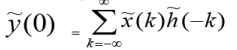= 1 * 0.5 + 0.5 * 1 = 1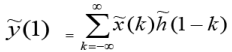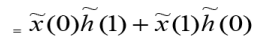= 1 * 1 + 0.5 * 0.5 = 1.25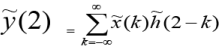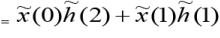1 * 0.5 + 0.5 * 1 = 1

~y (n) ={1, 1.25, 1, 1.25…..}

Q. Find Linear Convolution of same problem using DFT

Sol. The linear convolution will produce a 3-sample sequence. To avoid time aliasing we convert the 2-sample input sequence into 3 sample sequence by padding with zero.

For 3- point DFT

X (0) = 1.5           H (0) = 1.5

X (1) = 1+0.5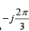H (1) = 0.5+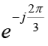X (2) = 1+0.5 e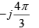H (2) = 0.5+ eY (K) = H (K) X (K)

Y (0) = 2.25

Y (1) = 0.5 + 1.25+ 0.5 eY (2) = 0.5 + 1.25+ 0.5 e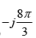Compute IDFT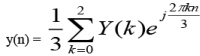y(0) = 0.5

y(1) =1.25

y(2) =0.5

y(n) = { 0.5, 1.25, 0.5} Ans

The document DFT (Frequency Domain Sampling) - Digital Signal Processing, Engineering Notes - Computer Science Engineering (CSE) is a part of Computer Science Engineering (CSE) category.
All you need of Computer Science Engineering (CSE) at this link: Computer Science Engineering (CSE)
 Use Code STAYHOME200 and get INR 200 additional OFF

Track your progress, build streaks, highlight & save important lessons and more!

,

,

,

,

,

,

,

,

,

,

,

,

,

,

,

,

,

,

,

,

,

,

,

,

;